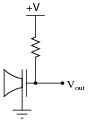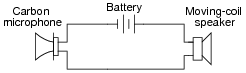# Learning Electronics

Learn to build electronic circuits

# Microphones

### Question 1:

Explain how a moving-coil, or dynamic microphone works. Identify how the principle of electromagnetic induction is put to practical use in this device.
A moving-coil microphone is built just the way the name suggests: a lightweight coil of wire gets moved past a magnet in proportion to vibrations in the air (sound), generating an AC voltage representative of the sound wave oscillations.
Follow-up question: characterize the typical output impedance of this microphone type. Is it generally considered a low-impedance or a high-impedance type of microphone? Why do you think this is?

Notes:
Ask your students where they located this information. it is important for students to learn where and how to research for needed information.

### Question 2:

Certain substances are known to physically deform (shorten or lengthen) when an electrical voltage is applied across their length. The effect is known as piezoelectricity. What types of substances are known to be piezoelectric? Identify some possible applications of this phenomenon.
Certain crystal substances, such as quartz, are known to be piezoelectric.

Notes:
As your students will no doubt discover in their research, piezoelectricity is a two-way effect: physical motion from electricity and visa-versa. It will be clear how well they did their research by the types of applications they think of for the ëlectricity-to-motion" form of piezoelectricity, given the typical physical scale of piezoelectric displacements.

### Question 3:

Explain how a crystal microphone works. Identify how the principle of piezoelectricity is put to practical use in this device.
Follow-up question: characterize the typical output impedance of this microphone type. Is it generally considered a low-impedance or a high-impedance type of microphone? Why do you think this is?
In a crystal microphone, a small piezoelectric crystal is stressed and unstressed by sound wave pressure, generating a voltage representative of the sound wave oscillations.

Notes:
Ask your students where they located this information. it is important for students to learn where and how to research for needed information.

### Question 4:

The basic circuit for an unamplified capacitor microphone looks like this:Vibrations in the air cause one metal plate to vibrate next to a stationary metal plate, rapidly changing the capacitance between the two plates. Explain how this simple circuit generates an AC voltage from this rapidly-varying capacitance, employing the mathematical relationship between charge (Q), capacitance (C) and voltage (V).
Given the equation Q = CV, we can see that changes in capacitance will either result in changes of stored charge for a given voltage (Q changes with V constant), changes in voltage for a given charge (V changes with Q constant), or changes in both Q and V. Remember that changes in electric charge over time ([dQ/dt]) is the mathematical definition of electric current (I).
Follow-up question: capacitor microphones are almost never used without some form of amplification. The voltage signal generated by the circuit shown in the question is simply too weak to use on its own. Explain why the signal is so weak.

Notes:
Ask your students where they located this information. it is important for students to learn where and how to research for needed information.

### Question 5:

An older microphone technology is the carbon microphone. Explain how this type of microphone worked, and why it was so simple to use in a circuit.
A carbon microphone generates a changing resistance representing sound wave oscillations. These microphones could be used as easily as this, with no amplifier circuit necessary:Notes:
Carbon microphones may represent an older, obsolete technology, but they are still illustrative for students.